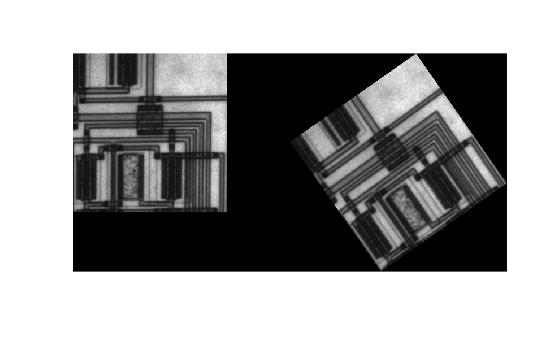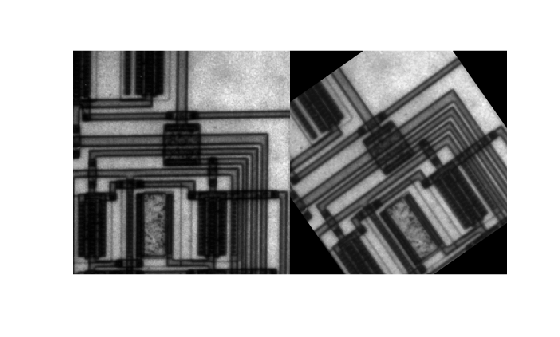Documentation

Rotate an Image

This example shows how to rotate an image using the imrotate function. When you rotate an image, you specify the image to be rotated and the rotation angle, in degrees. If you specify a positive rotation angle, the image rotates counterclockwise; if you specify a negative rotation angle, the image rotates clockwise.

By default, the output image is large enough to include the entire original image. Pixels that fall outside the boundaries of the original image are set to 0 and appear as a black background in the output image. You can, however, specify that the output image be the same size as the input image, using the 'crop' argument.

By default, imrotate uses nearest-neighbor interpolation to determine the value of pixels in the output image, but you can specify other interpolation methods. See the imrotate reference page for a list of supported interpolation methods.

Rotate an Image Counterclockwise

Read an image into the workspace.

Rotate the image 35 degrees counterclockwise. In this example, specify bilinear interpolation.

J = imrotate(I,35,'bilinear');

Display the original image and the rotated image.

figure
imshowpair(I,J,'montage')Crop a Rotated Image

Rotate the original image 35 degrees counterclockwise, specifying that the rotated image be cropped to the same size as the original image.

K = imrotate(I,35,'bilinear','crop');

Display the original image and the new image.

figure
imshowpair(I,K,'montage')